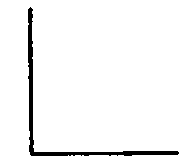An angle is the measure of the difference in direction of two lines. The lines are called sides, and the point of meeting, the vertex. The size of an angle is independent of the length of the lines.Fig. 40. Right Angle.Fig. 41. Acute Anglo.Fig. 42. Obtuse Angle.

If one straight line meets another (extended if necessary), Fig. 40, so that the two angles thus formed are equal, the lines are said to be perpendicular to each other and the angles formed are called right angles.

An acute angle is less than a right angle, Fig. 41.

An obtuse angle is greater than a right angle, Fig. 42.

## Surfaces

A surface is produced by the motion of a line; it has two dimensions - length and breadth.

A plane figure is a plane bounded on all sides by lines; the space included within these lines (if they are straight lines) is called a polygon or a rectilinear figure.